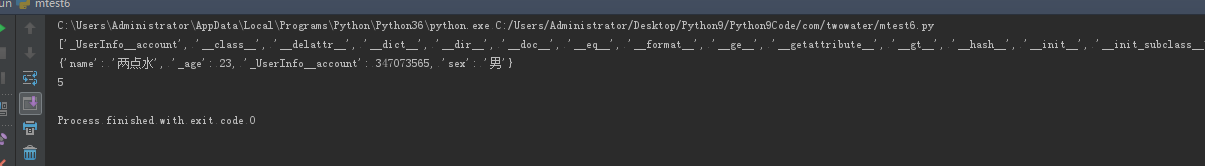# 七、类的继承

## 1、定义类的继承

``````class ClassName(BaseClassName):
<statement-1>
.
.
.
<statement-N>
``````

``````class ClassName(Base1,Base2,Base3):
<statement-1>
.
.
.
<statement-N>
``````

## 2、调用父类的方法

``````#!/usr/bin/env python
# -*- coding: UTF-8 -*-

class UserInfo(object):
lv = 5

def __init__(self, name, age, account):
self.name = name
self._age = age
self.__account = account

def get_account(self):
return self.__account

class UserInfo2(UserInfo):
pass

if __name__ == '__main__':
userInfo2 = UserInfo2('两点水', 23, 347073565);
print(userInfo2.get_account())

``````

## 3、父类方法的重写

``````#!/usr/bin/env python3
# -*- coding: UTF-8 -*-

class UserInfo(object):
lv = 5

def __init__(self, name, age, account):
self.name = name
self._age = age
self.__account = account

def get_account(self):
return self.__account

@classmethod
def get_name(cls):
return cls.lv

@property
def get_age(self):
return self._age

class UserInfo2(UserInfo):
def __init__(self, name, age, account, sex):
super(UserInfo2, self).__init__(name, age, account)
self.sex = sex;

if __name__ == '__main__':
userInfo2 = UserInfo2('两点水', 23, 347073565, '男');
# 打印所有属性
print(dir(userInfo2))
# 打印构造函数中的属性
print(userInfo2.__dict__)
print(UserInfo2.get_name())

``````## 4、子类的类型判断

``````#!/usr/bin/env python3
# -*- coding: UTF-8 -*-

class User1(object):
pass

class User2(User1):
pass

class User3(User2):
pass

if __name__ == '__main__':
user1 = User1()
user2 = User2()
user3 = User3()
# isinstance()就可以告诉我们，一个对象是否是某种类型
print(isinstance(user3, User2))
print(isinstance(user3, User1))
print(isinstance(user3, User3))
# 基本类型也可以用isinstance()判断
print(isinstance('两点水', str))
print(isinstance(347073565, int))
print(isinstance(347073565, str))

``````

``````True
True
True
True
True
False
``````